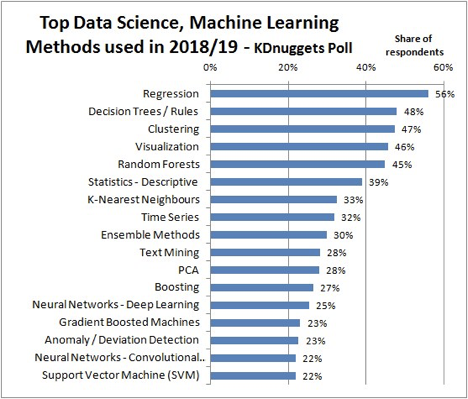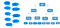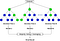# The Story of Random Forest

Mole’s Law claims the observation that the number of transistors in a dense integrated circuit (IC) doubles about every two years. After cumulating many years, the age of big data came in recent years with a sexy job, called Data Scientist. Although there is still no clear definition of what data scientists do, most of them played with a bunch of different models every day. But, what models do they commonly use? Here are the statistics in 2018/2019 from KDnuggests poll based on 833 voters.https://www.kdnuggets.com/2019/04/top-data-science-machine-learning-methods-2018-2019.html

Although some of you may doubt the data used on the graph above, for example, the size of the data might not be big enough to represent the data scientists, the models might be very different in different fields and some unseen factors in the survey might affect the result, we can still get some insights from the graph. Besides unsupervised learning (e.g. clustering, customer segment), what we could see is that the simple models which are easy to interpret (e.g. Regression) and tree-based models (e.g. Random Forests, Decision Trees) are on the top of the usage. In this article, I will explain the Random Forest in order to help someone who is interested in better understand the model.

# What is Random Forest?

Random forests are bagged decision tree models that split on a subset of features on each split and it can be used in both regression (continuous variables) and classification (discrete variables), or even a mix of the types of variables. It might be hard to understand this complex definition but let’s breakdown the significant terms to simplify the definition. So, what I will talk about are obviously the terms which seem hard to understand, “bagged” and “decision tree”, since nobody could avoid decision trees and “Bagged” methods in the stories of Random Forest. If we imagine the Random Forests as a real forest, the two concepts, “bagged” and “decision tree”, would be the roots of each tree.

# What is Decision tree?

Decision tree is like a tree that stands upside down. It breaks down the question we have by certain conditions which could be answered by Yes or No. Here is a conversation given by the book “Data Science From Scratch” and let’s see how we translate the conversation to the decision tree!From “Data Science From Scratch” by Joel Grus

In the example above, the decision tree is split on multiple features until we reach a conclusion of a certain animal, such as how the conversation made the decision that the animal is a lion. We named the blue boxes as nodes and black lines as branches. For the nodes, the first one is “Root nodes” and the one which would end your decision is “Terminal nodes/Leaves”. The question is, however, how can we make sure that our model would be not flexible since everyone would have their own styles of “tree” and a flexible model typically represents overfitting. For instance, in the first split, one would try to know if the animal lives in water firstly but another may want to know if the animal lives in North America. Therefore, the structure would be different with a very high probability, in other words, the decision tree would have high variances.

At each node, we have an optimization problem since we would like to gain as much information as we can and measure the quality of our split. For example, we minimize the residual sum of squares in regression problems and use Gini Impurity in classification problems.

Gini Impurity and how does it measure the quality of the splits?

“Gini Impurity (GI) is the probability of incorrectly classifying a randomly chosen element in the dataset if it were randomly labeled according to the class distribution in the dataset.” (Zhou)

where C is the number of classes and p(i) is the probability of randomly picking an element of class i.

Let’s assume we have 5 bottles of coconut sparkling water and another 5 bottles of cherry sparkling water. Before splitting, our G = p(1) * (1-p(1)) + p(2) * (1-p(2)) = 0.5 * (1–0.5) + 0.5 * (1–0.5) = 0.5. In most cases we split the two classes imperfectly, we have 4 cherry sparkling waters on the left side, 5 coconut sparkling waters and 1 cherry sparkling water on the right side. Now, we have G(left)=0 and G(right) = 1/6 * (1–1/6) + 5/6 * (1–5/6) = 0.278.

However, how can we measure the quality of the split quantitatively? Answer: Gini Gain! It’s calculated by the GI (before split) — GI(after split with weights on each branches). For example GI after split with weights in the sparkling water story is (0.4 * 0) + (0.6 * 0.278) = 0.167. So that Gini Gain = 0.5–0.167 = 0.333 which is not good since the higher Gini Gain is, the better split we have.

# What is Bagging (a.k.a Bootstrap Aggregating)?

The variability of the model is mainly from three parts, error, sample and how the trees were constructed, for example, decision trees have high variance. (explain) In order to reduce the high variance, we would use a powerful resampling method in statistics called Bootstrapping. Typically, we consider bootstrapping when we don’t have enough data because of some limitations of time or money. Theoretically, the basic idea is that we draw certain numbers randomly from all observations (maybe all features collected from a person) in our original dataset with replacement many times to get many corresponding subsets of our dataset. Then, we simply average them to estimate what we want (e.g. standard error). The idea that taking information from multiple models, such as averaging the results from many generated models, is called the ensemble method. It could help us reduce variability, sometimes get better predictions and better replicability.https://www.analyticsvidhya.com/blog/2020/05/decision-tree-vs-random-forest-algorithm/

“Bootstrapping is a statistical procedure that resamples a single dataset to create many simulated samples. This process allows for the calculation of standard errors, confidence intervals, and hypothesis testing” (Forst)

Now we apply this method to the decision trees. We created many decision trees that are trained on the corresponding bootstrapped training sets (each training set is a subset of our original dataset). Then the final prediction would be either calculated by averaging the values of many single decision trees (regression problem) or by the majority voting (classification problem). This bootstrap aggregating method which applies to decision trees is what we called Random Forest.

Let’s assume each result of the decision tree represents a student in a school. In the regression problem, we want to know the heights of students in the school. Nobody would choose the height of one student from the class to present the overall heights of students in the school. Therefore, the average heights of students in the school somehow better represent the overall heights of students in the school. (Sometimes what we would like to know is not numbers the heights.) The school would like to choose a president of students’ government from two candidates, as the U.S. presidential election. The candidate who has the majority votes will win the game.

# How cool is Random Forest?

• Handle main types of data: Random Forest could handle many different data types, such as categorical, binary, numerical, etc. It saves a lot of time to preprocess the data.
• Handle both regression and classification problems. Some machine learning algorithms can only be used in either regression or classification problems but Random Forest would handle both.
• Robust to outliers: It bins the outliers so that we don’t need to worry about the outlier issues in our Random Forest model.
• Moderate Variance and Low bias: We talked about the decision tree having extremely high variance and low bias but after we apply the bootstrap aggregating method, we only have moderate variance but keep the bias low.
• Non-parametric: The tree-based method is non-parametric so that it doesn’t require the data to follow a particular distribution.

# And…how bad is Random Forest?

• Heavy computation for large datasets: We would have tons of decision trees and determine a bunch of splits parallel so that it would be very slow if our dataset is big enough, even if CPU/GPUs work together.
• Interpretability: It may be hard to interpret this model to audiences without a certain background.
• Manipulation: It is like a black box so that many modelers have little control over what the model does.
• Overfitting: It can be easily overfitted so that we have to tune parameters(check out the article for tuning parameters in Python by towards data science if you like).

Tips: Improving your Random Forest model:

In this section, I would not cover the application of coding for random forest models since many excellent experts in data science talked about the applications in different fields with detailed coding step-by-step. However, I do recommend you check out these blogs(PYTHON, R) for coding applications. But in the coding implementation, one thing which you may spend much time on it is tuning hyperparameters. It is like you have to decide “how tall the trees are”, “how many trees you want in the forest” and “how fast you expect the forest to form”. You don’t know what is the “best” ( eg. lowest test RMSE/highest accuracy/lowest out-of-bag error/etc.) combination of these parameters before we run our model. Typically, the classic parameters in a random forest that we would like to tune, in Python, are how fast you want the machine learn (learning_rate), number of trees in the forest (n_estimator), max number of features used to split a node (max_feature), maximum number of levels in each tree (max_depth), minimum number of data points placed in a node before the node is split (min_samples_split), minimum number of data points allowed in a leaf node (min_samples_leaf) and method for sampling data points (with or without replacement) (bootstrap). We compare the train/validation/test scores together to make the decision which combination is the best one to be used in our final model. Usually, we might focus more on the test score since the performance of the model to the new points are more significant than the other two. This doesn’t mean, however, we accept bad performances in training and validation sets.

# References:

https://www.kth.se/social/upload/526eae35f27654034adf94ef/Moore's%20law%20-%2020131028.pdf

Forst, Jim. “Introduction to Bootstrapping in Statistics with an Example”. Statistics by Jim.https://statisticsbyjim.com/hypothesis-testing/bootstrapping/. Date accessed: June 17th, 2020

https://victorzhou.com/blog/gini-impurity

Data Science Graduate | Passion in data science | Modeling

## More from Haoda Song

Data Science Graduate | Passion in data science | Modeling